Saturday, June 12, 2021
Home > Latest Announcement > Real Life Scenario of Trigonometry

# Real Life Scenario of TrigonometryDownloading Link is given at Last

To know about the real life application of trigonometry, first we brief the introduction of the trigonometry. In school mathematics, we read that ‘TRIGONOMETRY’ is a combination of two Greek words ‘TRIGONO’ and ‘METRY’ as below in the figure.Thus, the trigonometry means the triangle measurement. Now, we know that a triangle has six parts; three angles and three sides. Therefore, the triangle measurement is actually the measurement of its sides and angles.Now, in the case of the right-angled triangles, there is a fixed relationship between the sides and the angles of the right-angled triangle. These fixed relationships are determined by the Trigonometry Ratios. Therefore, if at least the length of one side and the value of one angle are given, then all other angles and lengths can be determined.

But, for any triangle, the most use of trigonometry is in solution of triangles. If we know all the sides or two sides and the angle in between, or two angles and aside, then you can find all the angles and all the sides using trigonometry. These are the basis of trigonometry# Real Life Applications of Trigonometry

There are following real-life application of trigonometry:

Read More : 129 Maths Short Tricks

## In Measuring the Height and Building or Mountain

As we have studied that the trigonometry defines the relationship between sides and angles of a triangle. Thus, if we consider that building or mountain as the perpendicular and the base of the building is on the plane road.

Now, this building or mountain baseline is considered as the base of the triangle. At last, the line of sight is considered as the hypotenuse of the right-angled triangle. Then, this situation is considered as the application of a right-angled triangle.

Hence we can use the trigonometric function as sine, cosine, tangent, etc to find the height and distance of the building or mountain. Therefore, the measurement of the height and distance of the mountain or building is the real-life application of trigonometry.## In Aviation or Flight Engineering

As we know that when a flight r an airplane is flying in the sky then it is more affected by the wind. Thus, wind plays an important role in aviation technology.

The wind of flight direction is considered as the two perpendicular sides of a right-angled triangle in which the speed of wind and speed of the flight is measured in their direction.

Thus, by using the definition of trigonometrical ratios, we can easily calculate the direction of the destination. Therefore, flight engineering is the real-life application of trigonometry.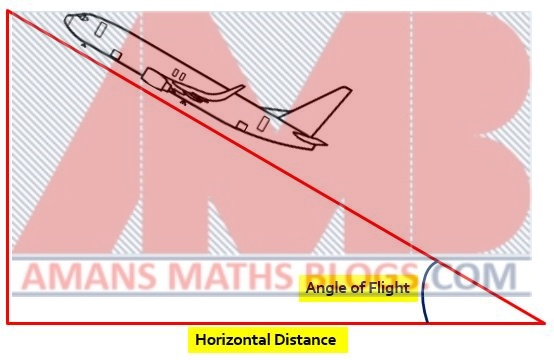## In Investigation of Crime Scene or Criminology

To investigate a crime, we need to identify the causes of any accidents, how the objects fall or what the angle is shot by the gun. All of these crimes are related to the angles and sides of the trajectory or triangle. Hence, this criminology is also considered as the real-life application of trigonometry.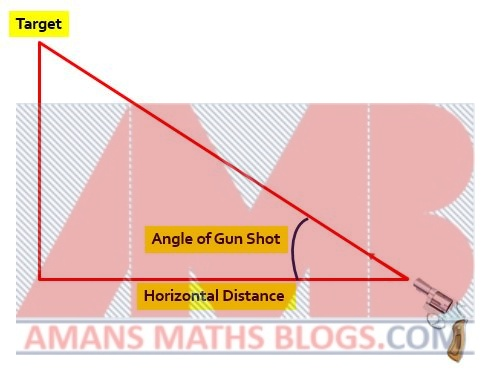## In Marine Biology

In marine biology, the biologist studies the marine life, that means it is the education of plants, animals and other organism that lives in the saltwater in the sea. Thus, marine life means sea life or ocean life.

Now, when biologist studies about marine life it always observes the animals and another organism etc in the water and their behavior, depth from water level, etc. All the sunlight and photosynthesis effects are studied.

Now, to measure the depth of the animal from the water level, it is considered as perpendicular to the right-angled triangle and sea level is considered as base of the right-angled triangle.

Hence, we can conclude the exact location of animals or another organism in the water from the surface of the water level by using the properties of a right-angled triangle or we can also use the concept of trigonometric ratios and its function.

Thus, this marine biologist is also considered as the real-life application of trigonometry.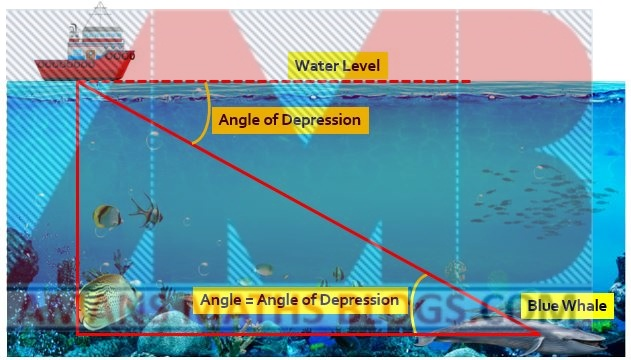## In Navigation

In navigation, we study about the process of monitoring and controlling the movement of craft or vehicle from a fixed location. it means, it is the process of determining the position and the direction of any moving bodies on or above the earth.

Thus, the navigation can be any type of marine navigation, land navigation, aeronautic navigation and space navigation. Now, to measure the exact location of these moving bodies, the compass and pinpoint poles are used that is based concept of trigonometric functions.

Hence, navigation is also the real-life application of trigonometry.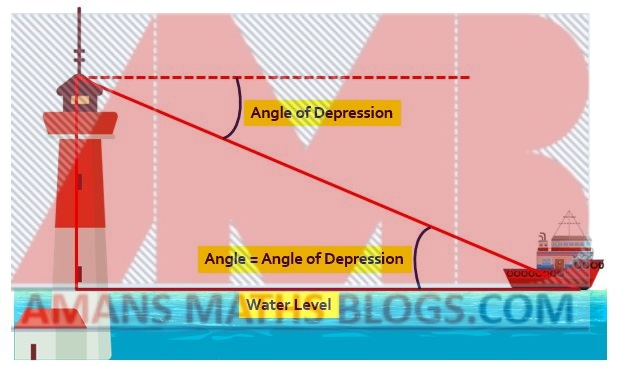## In Video Games

In gaming industries,  a very popular video game known as MARIO game, where the mario smoothly glide over the road blocks and uses a curve path or parabolic path to cross the obstacle.

This jump of mario developed by using the trigonometric functions. Hence, the video game development is also the real life application of trigonometry.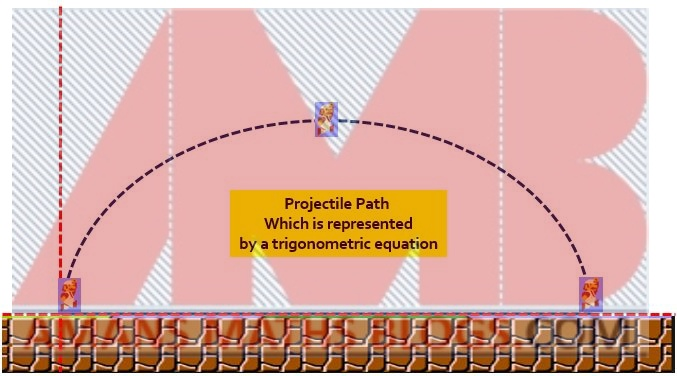## In Sound Waves or Light Waves

Sound waves travels in a repeating waves pattern and it is graphically represented by the sine and cosine curve of trigonometric function. Hence, the trigonometric function is very helpful for the study of sound waves and hence it is the real life application of trigonometry.## In Roof Inclination

In some buildings specially in bungalows, the roof of the house is inclined. This inclination of roof is measured by using the application of trigonometry function. Trigonometry defines the relationship between the sides of roofs and inclination of the roofs. Thus, the roof inclination in the buildings is also considered as the real life application of trigonometry.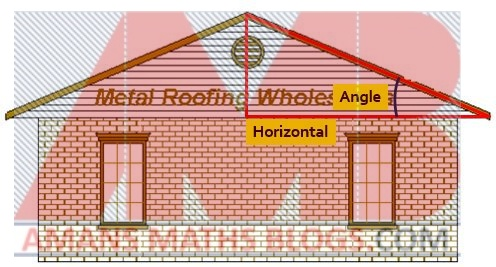## In Creation of Maps

In the creation of maps of any geographical location, we need to identify the distance and angle of path movement. Hence, it is closely related to the study to the study of triangle’s sides and angles measurement and hence it is also considered as the real-life application of trigonometry.## In Satellite

In the satellite system, we need to study the location and its position. Its location and position is measured by using the trigonometric function. Hence, it is also considered as the real-life application of trigonometry.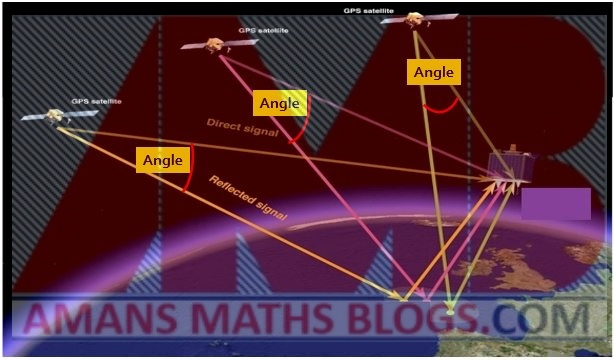## 2 thoughts on “Real Life Scenario of Trigonometry”

1.Danish Dakhwe says:

Real life examples are So simple to understand, You made it very easy to imagine how the trigonometry are being used in daily or real life.

1.AMAN RAJ says:

Thanks sir

error: Content is protected !!